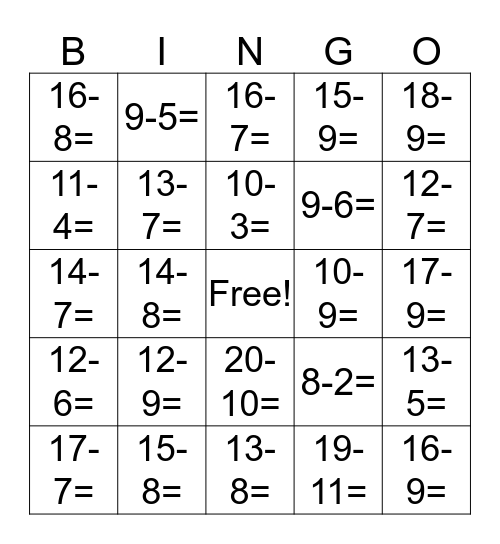# SubtractionThis bingo card has a free space and 24 words: 12-9=, 15-8=, 10-3=, 17-7=, 18-9=, 16-8=, 11-4=, 9-5=, 10-9=, 20-10=, 9-6=, 14-7=, 8-2=, 13-5=, 13-7=, 19-11=, 17-9=, 16-7=, 12-7=, 14-8=, 16-9=, 12-6=, 15-9= and 13-8=.

## Play Online